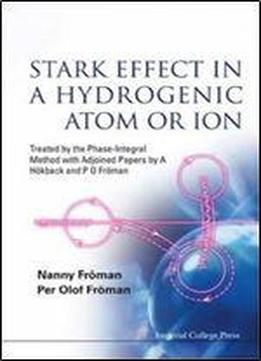# Stark Effect In A Hydrogenic Atom Or Ion by Nanny Froman / 2017 / English / PDF

This book treats the Stark effect of a hydrogenic atom or ion in a homogeneous electric field. It begins with a thorough review of previous work in this field since 1926. After the Schrodinger equation has been separated with respect to time dependence, centre of mass motion and internal motion, followed by a discussion of its eigenfunctions, the exact development in time of the probability amplitude for a decaying state is obtained by means of a formula analogous to the Fock Krylov theorem. From this formula one obtains by means of the phase-integral approximation generated from a particular base function non-relativistic formulas for profiles, energies and half-widths of the Stark levels. These formulas are then transformed into formulas expressed in terms of complete elliptic integrals. The formulas thus obtained are used for the calculation of energies and half-widths of 198 different Stark states, which are compared with the corresponding results obtained by other authors with the use of other methods. An analysis of this material indicates that the energy values obtained by the phase-integral method are at least as accurate as those obtained by other methods in more than half of the 198 cases. The book presents one of the most comprehensive asymptotic treatments of the Stark effect in atomic hydrogen that have been published. Contents: Schrodinger Equation, its Separation and its Exact Eigenfunctions Development in Time of the Probability Amplitude for a Decaying State Phase-Integral Method Derivation of Phase-Integral Formulas for Profiles, Energies and Half-Widths of Stark Levels Procedure for Transformation of the Phase-Integral Formulas into Formulas Involving Complete Elliptic Integrals Phase-Integral Quantities and Their Partial Derivatives with Respect to E and Z1 Expressed in Terms of Complete Elliptic Integrals. s

views: 594